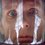# Pompeiu's theorem

Given an equilateral triangle ABC in the plane, and a point E in the plane of the triangle ABC, the lengths EA, EB, and EC form the sides of a (maybe, degenerate) triangle.Q.E.D.Note by Nicolae Sapoval
6 years, 11 months ago

This discussion board is a place to discuss our Daily Challenges and the math and science related to those challenges. Explanations are more than just a solution — they should explain the steps and thinking strategies that you used to obtain the solution. Comments should further the discussion of math and science.

When posting on Brilliant:

• Use the emojis to react to an explanation, whether you're congratulating a job well done , or just really confused .
• Ask specific questions about the challenge or the steps in somebody's explanation. Well-posed questions can add a lot to the discussion, but posting "I don't understand!" doesn't help anyone.
• Try to contribute something new to the discussion, whether it is an extension, generalization or other idea related to the challenge.

MarkdownAppears as
*italics* or _italics_ italics
**bold** or __bold__ bold
- bulleted- list
• bulleted
• list
1. numbered2. list
1. numbered
2. list
Note: you must add a full line of space before and after lists for them to show up correctly
paragraph 1paragraph 2

paragraph 1

paragraph 2

[example link](https://brilliant.org)example link
> This is a quote
This is a quote
    # I indented these lines
# 4 spaces, and now they show
# up as a code block.

print "hello world"
# I indented these lines
# 4 spaces, and now they show
# up as a code block.

print "hello world"
MathAppears as
Remember to wrap math in $$ ... $$ or $ ... $ to ensure proper formatting.
2 \times 3 $2 \times 3$
2^{34} $2^{34}$
a_{i-1} $a_{i-1}$
\frac{2}{3} $\frac{2}{3}$
\sqrt{2} $\sqrt{2}$
\sum_{i=1}^3 $\sum_{i=1}^3$
\sin \theta $\sin \theta$
\boxed{123} $\boxed{123}$

Sort by:

Very nice :D

- 6 years, 11 months ago

I think some description would be more helpful! :)

- 6 years, 11 months ago

Look for corresponding congruent parts of the red triangle and the original blue segments. Also note that bit in the bottom left that shows an angle of measure 60 degrees.

- 6 years, 11 months ago

I know this theorem well enough just as you do.. But those who haven't even heard of this would need some description I think..

- 6 years, 11 months ago

Given $\Delta ABC$ and the point $E$. Now, using $CA$ as base, construct an equilateral $\Delta ADC$. The position of the point $E'$ with respect to vertices $A$,$D$ and $C$ of $\Delta ACD$ is same as the that of point $E$ with respect to vertices $A$, $C$ and $B$ respectively of $\Delta ABC$. Let $\angle EAB = \theta$. Therefore, $\angle E'AC = \theta$ and $\angle CAE = 60-\theta$. Hence, $\angle E'AE = 60^\circ$ . And $\Delta E'AE$ is an equilateral triangle. Therefore, $EE' = EA$. Also, $E'C = EB$ because of our construction. Therefore, we have constructed a degenerate $\Delta CE'E$ with side lengths $CE'=EB$ , $E'E=EA$ and $EC$ which was to be proved.

- 6 years, 11 months ago

The only problem with the solution is that it works only for points within the $\Delta ABC$. A separate proof is required for points outside the triangle.

- 6 years, 11 months ago

q.e.d

- 6 years, 11 months ago

Can't really understand, can someone please tell me :3

- 6 years, 11 months ago

Basically he rotated the whole triangle about A by 60 degrees. A lot of length equalities follow and we get a triangle with sides equal in length to EA, EB, and EC.

- 6 years, 11 months ago

I agree with Snehal.

- 6 years, 11 months ago

What is a 'degenerate' triangle?

- 6 years, 11 months ago

A triangle whose vertices are collinear.

- 6 years, 11 months ago

Please explain degenerate and Q.E.D and what is the use of this theorem ...... Please i 'm weak at maths

- 6 years, 11 months ago

QED is latin "quod erat demonstrandum"; it means 'Which was to be demonstrated'; other forms include "Hence proved", "Which Was What Was Wanted ($\text{W}^5$)",etc. Sometimes, as a joke "Quite easily done". You can read the full article. Degenerate: A triangle with all points on the same line.

- 6 years, 11 months ago

excellent.................

- 6 years, 11 months ago

2

- 6 years, 11 months ago